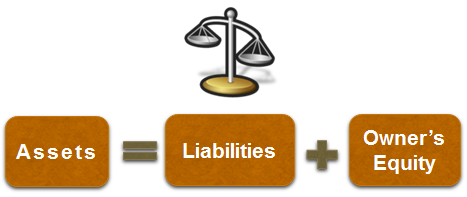# The Accounting Equation

Businesses exchange items of equal value, real or perceived. Imagine that an exchange is like balancing a scale: the left side goes down (a service is given) and the right side reacts (cash is received) to maintain the balance of the scale.

Accounting uses a technique to show how a transaction changes the business's resources while maintaining a balance, or showing the equal value of the exchange. This is called the accounting equation.

The accounting equation is a simple tool that helps us understand how various transactions affect the elements of a financial statement, i.e., the asset, liability, and owner's equity accounts, and how these accounts are related to each other.

### What is the Accounting Equation?

The accounting equation is presented as Assets = Liabilities + Owner’s EquityNote that in case of a corporation, instead of owner’s equity we have shareholder’s equity as many shareholders invest money and have equity stake in the corporation.

This equation will always be balanced. So, if the business has assets worth $80,000, and owner’s equity of$20,000, then we can say that the liabilities are equal to $60,000.$80,000 (Assets) = $60,000 (Liabilities) +$20,000 (Equity)